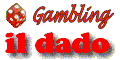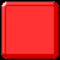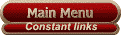Feature article - General Gambling
 The Probability-Based Strategy, by Catalin Barboianu Probability Theory is the only rigorous theory modeling hazard, so any gambling strategy must be probability based. This statement is the subject of my article below, which is also part of my last book, "Probability Guide of Gambling". This guide holds a large collection of probability results and strategies, covering thousands of gaming situations from all major games including dice, slots, baccarat, roulette, blackjack, poker, electronic poker, lottery and sports bets. It is not a classical scientific study, but a manifold application in 'practical guide' form. It is structured so gamblers with minimal mathematical background can skip the mathematical parts and directly find the results they need. In this guide, the presented numerical results are accompanied where considered necessary by recommendations to choose certain gaming variants or even certain games. These recommendations are built on the 'probability based strategy' and are stated within the context of the personal criteria of each player, regarding the goal of the game, the process of game and the player's risk profile. What does a probability based strategy actually mean? "A strategy using criteria of evaluation and comparison of probabilities of various gaming events, in making decisions for accomplishing the proposed goal", might do for a general definition. In most cases the declared goal of the gambler is immediate winning of cash, but also fun, entertainment or experiencing certain emotions that are specific to competition or risk might be his or her goals. Here we will show why the probability based strategy is optimal in situations where the goal of the player is the winning cash. As we said, a probability based strategy is using decisions made as result of evaluating the probability figures. These are decisions such as making a particular playing decision at a certain moment of the game and also of choosing a certain game to begin with. Probability Theory is the only rigorous theory that models the hazard. But it only offers measurements of this hazard and not certainties about punctual events. The certainty offered by the "Law of Large Numbers" (see page 35 of my book) is one of the limit, approximation and existence type. This theorem does not provide precise information about occurrence of expected events (for example, it cannot tell how many times we have to throw a die to surely get a 5), but even the limit behavior gives additional information. The basic element of probability based strategy is choosing the gaming variant that offers the highest probability of occurrence of expected event, in condition of identical ulterior advantages. Assume that the player has reached a decision situation, at a certain game. His or her options are the gaming variants A and B, both providing the same ulterior advantages, in case of occurrence of expected event. Why is it good for the player to choose the gaming variant that offers the higher probability of expected event, as long as Probability Theory does not provide him or her any certainty about it? If we answer this question, the choice of probability based strategy as being optimal will be justified. Let's denote by P(A) the probability of occurrence of expected event in experiment A (playing variant A) and by P(B) the probability of occurrence of expected event in experiment B (playing variant B) and assume that P(A)> P(B). We will first provide a motivation for choosing the gaming variant A, in case the gambler is a regular player of this game. Let's come back to the "Law of Large Numbers". This theorem states that, in a sequence of independent experiments, the relative frequency of occurrence of a certain event is converging towards the probability of that event. Let's consider experiment A as being part of a sequence of experiments, namely the sequence of experiments of playing variant A by the same gambler, at the same type of game, every time he or she reaches that respective situation (in ulterior games). Similarly, let's consider experiment B as being part of the sequence of analogue experiments where the gambler chooses variant B (hypothetically). Let's denote by a(n) the number of occurrences of expected event after n experiments of A type and by b(n) the number of occurrences of expected event after n experiments of B type. We will show that a sufficiently big number N exists, such that a(n) > b(n), for any n > N. The demonstration is obvious: We assume the opposite, namely that for any N, exists n > N such that a(n) < b(n) or a(n) = b(n). In the same conditions, results a(n)/n < b(n)/n or a(n)/n = b(n)/n. By passing to the limit in this inequality and using the law of large numbers (a(n)/n --> P(A), b(n)/n --> P(B)), we get that P(A) < P(B) or P(A) = P(B) and that is false (we initially assumed that P(A) > P(B)). Therefore, a(n) > b(n) from a certain rank upward, within the sequence of experiments. This means that the number of favorable events will be bigger (cumulatively) in case of sequence of experiments of A type, from a certain rank upward. Although no mathematical result establishes which this number N is (we only know it exists), the above demonstration is offering a motivation (purely theoretical) for choosing the gaming variant A, namely the following: "We know there is a level N from which the cumulative number of favorable events is bigger in the situation of a sequence of experiments of A type. If we "alter" this sequence by introducing experiments of B type, the fulfillment of the N experiments will be delayed." This is the only theoretical motivation that uses probability properties. Because we do not have information about N, which can be any size and eventually never reached, the motivation remains a theoretical one and might have no practical coverage, except the cases in which the difference P(A) - P(B) is significant. Despite all these, it remains a motivation that justifies the choice of probability based strategy. But how do we justify the choice of probability based strategy in a situation where the gambler is not a regular player of the particular game, he or she plays it only once so the time tendency is not a motivation any more? We have here a punctual gaming situation, in which the gambler has to make a decision to choose one of variants A and B, in a condition of identical ulterior advantages. Why should he or she choose variant A, which offers a higher probability for the expected event? A similar situation, not apparently related to gambling, is the following: You are in a phone box and you must urgently communicate important information to one of your neighbors. (Let's say you left your front door open). You have only one coin, so you can make one single call You have two neighboring houses Two persons are living in one of them and three persons are living in the other Both their telephones have answering machines. Which one of the two numbers will you call? Isn't it true that you "feel" that you must choose the house with 3 persons, because the probability for someone to be at home is higher? But how do we rigorously explain this optimal choice, based on probability criteria? Coming back to the assumed gaming situation, the "Law of Large Numbers" will still provide us a theoretical motivation for the choice. Although we don't have here a sequence of tangible experiments, not even expected ones (as in the regular player case) to include respective experiment, we still can introduce it in such a sequence, so as for application of the "Law of Large Numbers" to be available. Let's consider the sequence of independent experiments of all experiments of A type performed in time by other players, in the same gaming situation, chronologically, until the respective gaming moment. Similarly, let's consider the sequence of experiments of B type, chronologically performed until the respective moment. For the two previously defined sequences of experiments we can do the same deduction as in the first case (regular player), based on the law of large numbers, and the result will be: a sufficiently big number N of experiments exists, such that for any n > N, we have a(n) > b(n) or a(n) = b(n). Therefore, the motivation of "not altering" the sequence of experiments of A type still stands valid, even if only at the theoretic level. So, the answer to the question generated by the your required choice is the following: "Although we don't know where the rank N (provided by the law of large numbers) is standing and also if experiment A offers a favorable result, it is proper to choose the gaming variant A at least for the reason of other eventual ulterior similar gaming situations to form an "unaltered" sequence of experiments of A type." As we said, the motivation is a purely theoretical one, but it accomplishes the proposed goal, namely to show that the probability based strategy is optimal. The above presented situation of an isolated game has a much smaller practical coverage than in the case of a regular player, except the cases in which the difference P(A) - P(B) is big enough. The entire demonstration above was done in a theoretical situation where the favorable event expected after each gaming variant offers the same advantage to the player. This advantage could be the immediate winning of cash, a superior position in the game, or other strategic advantages. A probability based strategy can be seen as optimal only after taking into account the player's goals and these could be various. Thus, the probability criteria do not always determine decisions, but also the personal and subjective criteria of the player. As is well known, probabilities do not provide certainties about hazard. The probability based strategy is optimal, but it still does not guarantee winning. In other words, such a strategy is the optimum strategy to adopt when trying to "push your luck", but it does not bring you luck made to order. Although the probability of getting 1 after one die throw is 1/6, if we throw the die 6 times, this does not assure us getting a 1, just as throwing the die 10 times does not assure it. Theoretically, is possible to throw the die 1,000 times and not get a single 1, although it is improbable. The only thing we know for sure is that the frequency of occurrences of 1 is getting closer to 1/6, as the number of throws increases. There is an obvious difference between the terms "possible" and "probable". Probability is a measure, mathematically rigorously defined, whereas "possible" is a much more complex and difficult to define philosophical category. Probability is modeling a minute part of the possible. A hypothetical rigorous definition of "possible" should include another "zero degree" philosophical term, namely the reality. This dimension difference between the two terms is the most relevant expression of the fact that we cannot rule over the hazard, but we can "push our luck" by using the laws of probability, even if this often results in losses. Catalin Barboianu is also the author of "Probability Guide of Gambling" book. [Article submitted June 2004] Gambling Articles (Main Index) Great gambling information site Use the "Main Menu" on the top right margin to explore this site. This is a comprehensive gambling information site with advice on winning, how to gamble, Betting systems, gambling articles, world land-based casinos directory, casino reviews (reviewed and rated), the best Online casinos, free online games, and lots of gamblers information and resources. Topics covered include game rules, how to play, how to win, betting strategies, gambling tips, glossary, on: Blackjack, Roulette (showing Table layouts of American, European and French roulettes and also the fast-play Roulite version), Poker classic and variants including Texas hold'em and Let-it-ride, Craps (dice game), Jackpot slots, Videopoker, Video games, Baccarat, Keno, Lotto/Lottery, Powerball, Bingo, Sports betting, Horse and Greyhound racing (including racetracks, race programs and results), and new gambling games. This is the gambling website with lots of information and resources as well as helpful advice and frequent updates thanks to your helpful feedback. Gambling Articles (Main Index)Advice Resources Facilities Home ¬ Start here! All about...